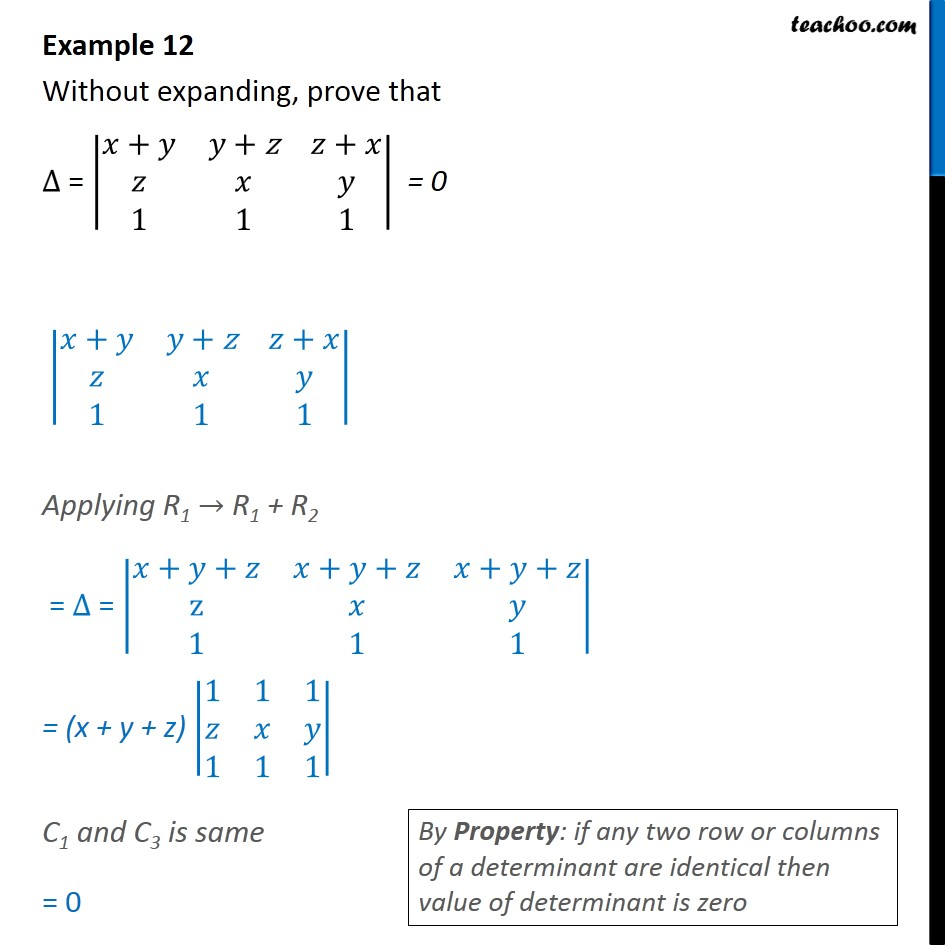1. Chapter 4 Class 12 Determinants
2. Concept wise
3. Two rows or columns same

Transcript

Example 12 Without expanding, prove that = + + z + 1 1 1 = 0 + + z + 1 1 1 Applying R1 R1 + R2 = = + + + + + + z 1 1 1 = (x + y + z) 1 1 1 1 1 1 C1 and C3 is same = 0

Two rows or columns same